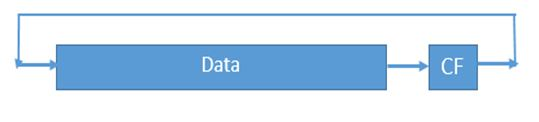# Shift and Rotate Instructions in 8086 Microprocessor

In this article, we are going to study another type of instructions of the 8086 microprocessor which are used for shifting or rotating the contents of the register. We will first discuss the working of each of these instructions and will then also discuss the syntax for each of them.
Submitted by Monika Sharma, on July 29, 2019

As we know that any machine (system) works on machine language, which consists of binary numbers. In the 8086 microprocessor, we have 16-bit registers to handle our data. Sometimes, the need to perform some necessary shift and rotate operations on our data may occur according to the given condition and requirement. So, for that purpose, we have various Shift and Rotate instructions present in the 8086 microprocessor. Let us discuss them one by one and understand their working:

1. SHR : Shift Right
2. SAR : Shift Arithmetic Right
3. SHL : Shift Left
4. SAL : Shift Arithmetic Left
5. ROL : Rotate Left
6. ROR : Rotate Right
7. RCL : Rotate Carry Left
8. RCR : Rotate Carry Right

### 1) SHR : Shift Right

The SHR instruction is an abbreviation for ‘Shift Right’. This instruction simply shifts the mentioned bits in the register to the right side one by one by inserting the same number (bits that are being shifted) of zeroes from the left end. The rightmost bit that is being shifted is stored in the Carry Flag (CF).

```Syntax: 	SHR Register, Bits to be shifted
Example:	SHR AX, 2
```

Working: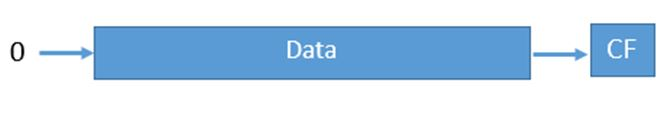### 2) SAR : Shift Arithmetic Right

The SAR instruction stands for ‘Shift Arithmetic Right’. This instruction shifts the mentioned bits in the register to the right side one by one, but instead of inserting the zeroes from the left end, the MSB is restored. The rightmost bit that is being shifted is stored in the Carry Flag (CF).

```Syntax: 	SAR Register, Bits to be shifted
Example:	SAR BX, 5
```

Working: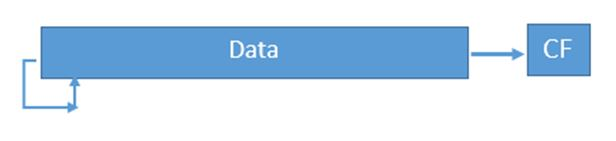### 3) SHL : Shift Left

The SHL instruction is an abbreviation for ‘Shift Left’. This instruction simply shifts the mentioned bits in the register to the left side one by one by inserting the same number (bits that are being shifted) of zeroes from the right end. The leftmost bit that is being shifted is stored in the Carry Flag (CF).

```Syntax: 	SHL Register, Bits to be shifted
Example:	SHL AX, 2
```

Working: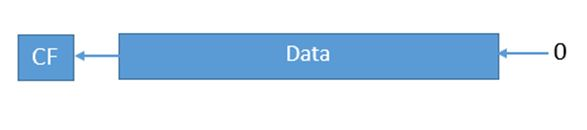### 4) SAL : Shift Arithmetic Left

The SAL instruction is an abbreviation for ‘Shift Arithmetic Left’. This instruction is the same as SHL.

```Syntax: 	SAL Register, Bits to be shifted
Example:	SAL CL, 2
```

Working: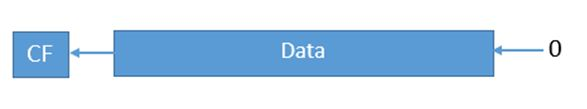### 5) ROL : Rotate Left

The ROL instruction is an abbreviation for ‘Rotate Left’. This instruction rotates the mentioned bits in the register to the left side one by one such that leftmost bit that is being rotated is again stored as the rightmost bit in the register, and it is also stored in the Carry Flag (CF).

```Syntax: 	ROL Register, Bits to be shifted
Example:	ROL AH, 4
```

Working: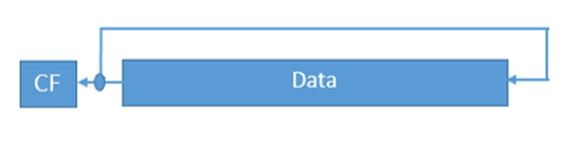### 6) ROR : Rotate Right

The ROR instruction stands for ‘Rotate Right’. This instruction rotates the mentioned bits in the register to the right side one by one such that rightmost bit that is being rotated is again stored as the MSB in the register, and it is also stored in the Carry Flag (CF).

```Syntax: 	ROR Register, Bits to be shifted
Example:	ROR AH, 4
```

Working: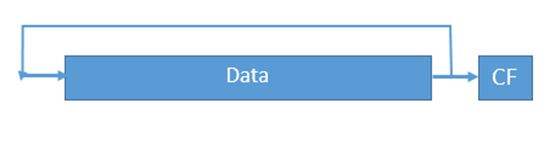### 7) RCL : Rotate Carry Left

This instruction rotates the mentioned bits in the register to the left side one by one such that leftmost bit that is being rotated it is stored in the Carry Flag (CF), and the bit in the CF moved as the LSB in the register.

```Syntax: 	RCL Register, Bits to be shifted
Example:	RCL CH, 1
```

Working: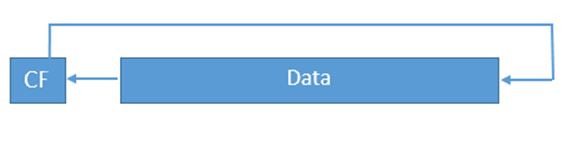### 8) RCR : Rotate Carry Right

This instruction rotates the mentioned bits in the register to the right side such that rightmost bit that is being rotated it is stored in the Carry Flag (CF), and the bit in the CF moved as the MSB in the register.

```Syntax: 	RCR Register, Bits to be shifted
Example:	RCR BH, 6
```

Working: